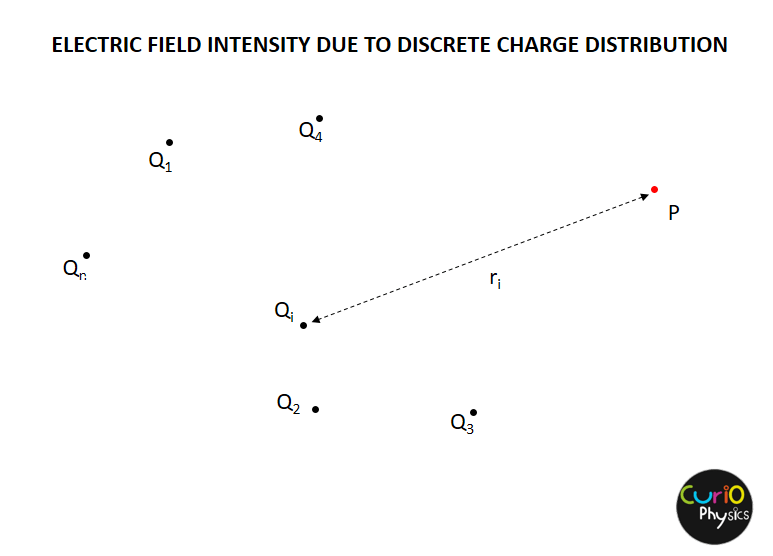# Electric Field Intensity due to Continuous Charge Distribution

##### ELECTRIC FIELD INTENSITy due to continuous CHARGE DISTRIBUTIONS

Electric Field Intensity due to Continuous Charge Distribution :- With the help of Coulomb’s Law and Superposition Principle, we can easily find out the Electric Field Intensity due to a system of charges. In this article we are going to find out electric field intensity due to a discrete charge distribution and a continuous charge distribution.

##### Electric Field Intensity Due to discrete charge distribution

Suppose there are n charge particles present in a region and we have to find out net electric field intensity at a point P. Then first we have to find the electric field intensity due to individual charge particles and after that we have to take the vector sum of Electric Field Intensity due to individual charge particles.Electric field intensity due to Qi at point P$\displaystyle {{{\vec{E}}}_{P{{Q}_{i}}}}=k\frac{{{Q}_{i}}}{{{r}_{i}}^{2}}\hat{n}$

By principle of superposition net electric field due to whole distribution of charge :-$\displaystyle {{{\vec{E}}}_{net}}=\sum\limits_{i=1}^{i=n}{{{{\vec{E}}}_{P{{Q}_{i}}}}}$

##### Electric Field Intensity due to Continuous Charge Distribution

As we know that continuous charge distribution is of three types :-

1. Linear Charge Distribution
2. Surface Charge Distribution
3. Volume Charge Distribution

So let us find Intensity of field due to them one by one….

1. Linear Charge Distribution :-

Let us consider charge distribution is linear (like on a straight line or at the circumference of a circle) with linear charge density λ. To determine Electric Filed Intensity at a point, let us first find force experienced by a test charge q0 placed at that point due to this distribution.

Let us take a small element MN of length dl on the line. Charge on this element dq = λdl [$\displaystyle \because \lambda =\frac{dq}{dl}$].So force on q0 at P due to small element MN,$\displaystyle d\vec{F}=\frac{{{q}_{0}}}{4\pi {{\varepsilon }_{0}}}\frac{dq}{{{r}^{2}}}\hat{r}=\frac{{{q}_{0}}}{4\pi {{\varepsilon }_{0}}}\frac{\lambda dl}{{{r}^{2}}}\hat{r}$

∴ Total force on q0 due to line charge distribution is$\displaystyle \vec{F}=\frac{{{q}_{0}}}{4\pi {{\varepsilon }_{0}}}\int\limits_{L}{\frac{\lambda }{{{r}^{2}}}\hat{r}dl}$

Finally as we know Electric Filed Intensity is given by$\displaystyle \vec{E}=\frac{{\vec{F}}}{{{q}_{0}}}$, hence$\displaystyle \vec{E}=\frac{1}{4\pi {{\varepsilon }_{0}}}\int\limits_{L}{\frac{\lambda }{{{r}^{2}}}}dl\hat{r}$

If linear charge density λ is constant, then$\displaystyle \vec{E}=\frac{\lambda }{4\pi {{\varepsilon }_{0}}}\int\limits_{L}{\frac{dl}{{{r}^{2}}}}\hat{r}$

2. Surface Charge Distribution :-

Let us consider that charge is distributed continuously on a surface (like a sheet) and surface charge density is σ. In this case also, to find Electric Filed Intensity at a point, let us first find force experienced by a test charge q0 placed at that point.

Let us take a small area element of area dS on the sheet. Charge on this area element dq = σdS [$\displaystyle \because \sigma =\frac{dq}{dS}$].So force on q0 at P due to small element of area dS,$\displaystyle d\vec{F}=\frac{{{q}_{0}}}{4\pi {{\varepsilon }_{0}}}\frac{dq}{{{r}^{2}}}\hat{r}=\frac{{{q}_{0}}}{4\pi {{\varepsilon }_{0}}}\frac{\sigma dS}{{{r}^{2}}}\hat{r}$

∴ Total force on q0 due to surface charge distribution is$\displaystyle \vec{F}=\frac{{{q}_{0}}}{4\pi {{\varepsilon }_{0}}}\int\limits_{S}{\frac{\sigma }{{{r}^{2}}}\hat{r}dS}$

Finally, Electric Filed Intensity,$\displaystyle \vec{E}=\frac{{\vec{F}}}{{{q}_{0}}}$

⇒$\displaystyle \vec{E}=\frac{1}{4\pi {{\varepsilon }_{0}}}\int\limits_{S}{\frac{\sigma }{{{r}^{2}}}}dS\hat{r}$

If surface charge density σ is constant, then$\displaystyle \vec{E}=\frac{\sigma }{4\pi {{\varepsilon }_{0}}}\int\limits_{S}{\frac{dS}{{{r}^{2}}}}\hat{r}$

3. Volume Charge Distribution :-

Let us consider that charge is continuously distributed in a volume (like in a sphere or cube) and volume charge density is ρ.

Let us take a small volume element of volume dV in the sphere. Charge on this volume element dq = ρdV [$\displaystyle \because \rho =\frac{dq}{dV}$].Force on q0 at P due to small element of volume dV,$\displaystyle d\vec{F}=\frac{{{q}_{0}}}{4\pi {{\varepsilon }_{0}}}\frac{dq}{{{r}^{2}}}\hat{r}=\frac{{{q}_{0}}}{4\pi {{\varepsilon }_{0}}}\frac{\rho dV}{{{r}^{2}}}\hat{r}$

∴ Total force on q0 due to volume charge distribution is$\displaystyle \vec{F}=\frac{{{q}_{0}}}{4\pi {{\varepsilon }_{0}}}\int\limits_{V}{\frac{\rho }{{{r}^{2}}}\hat{r}dV}$

Electric Filed Intensity,$\displaystyle \vec{E}=\frac{{\vec{F}}}{{{q}_{0}}}$

⇒$\displaystyle \vec{E}=\frac{1}{4\pi {{\varepsilon }_{0}}}\int\limits_{V}{\frac{\rho }{{{r}^{2}}}}dV\hat{r}$

If volume charge density ρ is constant, then$\displaystyle \vec{E}=\frac{\rho }{4\pi {{\varepsilon }_{0}}}\int\limits_{V}{\frac{dV}{{{r}^{2}}}}\hat{r}$